Courses

# General Solution To Second Order Homogeneous LTI System Mechanical Engineering Notes | EduRev

## Mechanical Engineering : General Solution To Second Order Homogeneous LTI System Mechanical Engineering Notes | EduRev

The document General Solution To Second Order Homogeneous LTI System Mechanical Engineering Notes | EduRev is a part of the Mechanical Engineering Course Theory of Machines (TOM).
All you need of Mechanical Engineering at this link: Mechanical Engineering

General Solution to Second-Order Homogeneous LTI System

We now focus on the zero input response of the second-order LTI system of Eq. (1–17), i.e., we focus on the system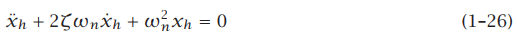Suppose that we guess the solution to Eq. (1–26) as

xh(t) = eλt                                                                                                           (1–27)

where λ is constant that has yet to be determined. Differentiating the assumed solution of Eq. (1–27) twice, we have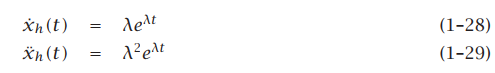Substituting the results of Eqs. (1–28) and (1–29) into (1–26), we obtain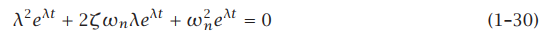Then, because eλt  is not zero as a function of time, it can be dropped from Eq. (1–30) to give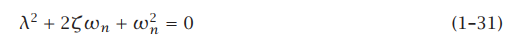Equation (1–31) is called the characteristic equation whose roots give the behavior of the zero input response of Eq. (1–17). Using the quadratic formula, the roots of Eq. (1–31) are given as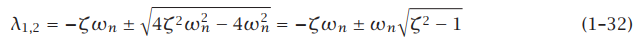It can be seen that the types of roots admitted by Eq. (1–31) depend upon the value of ζ. In particular, the types of roots are governed by the quantity ζ2 − 1. We have three cases to consider: (1) 0 ≤ ζ < 1, (2) ζ = 1, and (3) ζ > 1. We now consider each of these cases in turn.

Case 1: 0 ≤ ζ < 1 (Underdamping)

When 0 ≤ ζ < 1 the zero input response is said to be underdamped. For an underdamped system the quantity ζ2 − 1 < 0 which implies that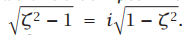The roots of the characteristic equation for an underdamped system are then given as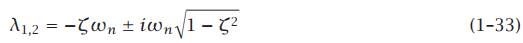It is seen from Eq. (1–33) that the roots of the characteristic equation for an underdamped system are complex. Furthermore, the general zero input response for an underdamped system is given as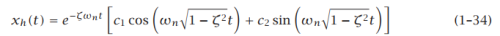Eq. (1–34) can be written as

xh(t) = e −ζωnt (c1 cos ωdt + c2 sinωdt)                                                                  (1–35)

where the quantity ωd = ω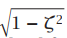is called the damped natural frequency of the system. The constants c1 and c2 can be solved for by using the initial conditions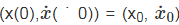as follows. First, substituting the initial condition x(0) = x0 into Eq. (1–35), we obtain c1 as

xh(0) = x= c1                                                                                                     (1–36)

Next, differentiating xh(t) in Eq. (1–35), we obtain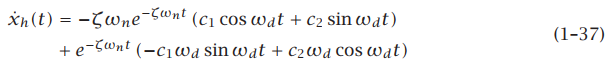Applying the initial condition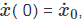we obtain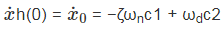(1–38)

Substituting the result for c1 from Eq. (1–36) into Eq. (1–38), we obtain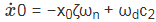(1–39)

Solving for c2 we have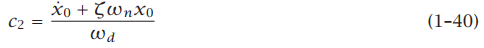The zero input response for an underdamped system is then given as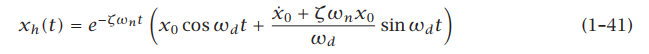A schematic of the underdamped zero input response for various values of 0 ≤ ζ < is shown in Fig. 1–2.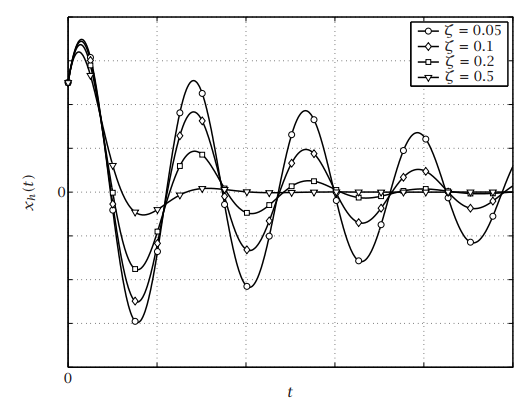Figure 1–2 Schematic of the zero input response of an underdamped second-order linear time-invariant system.

Case 2: ζ = 1 (Critical Damping)

When ζ = 1 the zero input response is said to be critically damped. For critically damped system the quantity ζ2 − 1 = 0 which implies that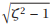= 0. The roots of the characteristic equation for an underdamped system are then given as

λ1,2 = −ζωn = −ωn                                                                        (1–42)

It is seen from Eq. (1–42) that the roots of the characteristic equation for a critically damped system are real and repeated (i.e., the two roots are the same). Furthermore, the general zero input response for a critically damped system is given as

xh(t) = e −ωnt (c1 + c2t)                                                                (1–43)

The constants c1 and c2 can be solved for by using the initial conditions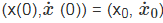as follows. First, applying the initial condition x(0) = x0 into Eq. (1–43), we have

xh(0) = x0 = c                                                                              (1–44)

Next, differentiating Eq. (1–43), we obtain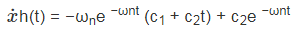(1–45)

Applying the initial condition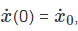we obtain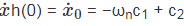(1–46)

Substituting the result for c1 from Eq. (1–44), we have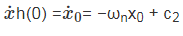(1–47)

Solving Eq. (1–47) for c2 gives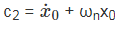(1–48)

The zero input response for an critically damped system is then given as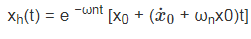(1–49)

A schematic of a critically damped zero input response is shown in Fig. 1–3.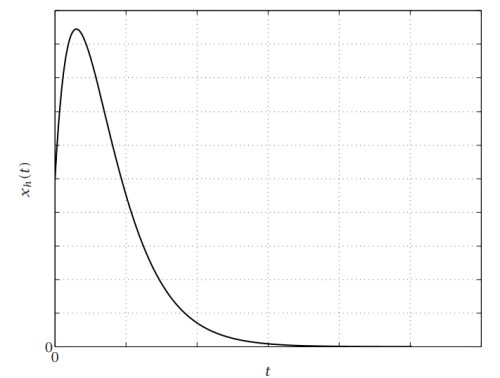Figure 1–3      Schematic of the zero input response of a critically damped second-order linear time-invariant system.

Case 3: ζ > 1 (Overdamping)

When ζ > 1 the zero input response is said to be overdamped. For an overdamped system the quantity ζ2 −1 > 0 which implies that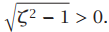The roots of the characteristic equation for an underdamped system are then given as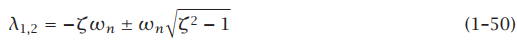It is seen from Eq. (1–50) that the roots of an overdamped system are real and distinct. Furthermore, the general zero input response for an overdamped system is given as

xh(t) = c1eλ1t + c2eλ2t                                                                                          (1–51)

The constants c1 and c2 can be solved for by using the initial conditions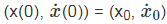as follows. First, applying the initial condition x(0) = x0, we obtain

xh(0) = x0 = c1 + c2                                                                                             (1–52)

Next, differentiating Eq. (1–51) gives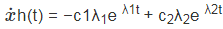(1–53)

Then, applying the initial condition x( ˙ 0) = x˙0, we obtain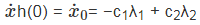(1–54)

Equations (1–52) and (1–54) can then be solved simultaneously for c1 and c2 to give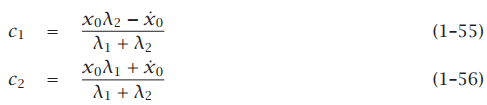The general zero input response for an overdamped system is then given as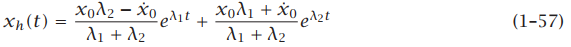A schematic of an overdamped zero input response for various values of ζ > 1 is shown in Fig. 1–4.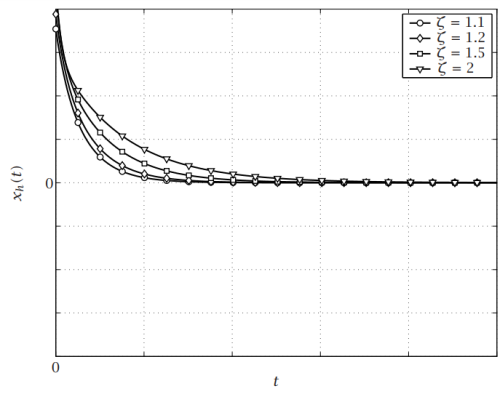Figure 1–4 Schematic of the zero input response of an overdamped second-order linear time-invariant system.

Offer running on EduRev: Apply code STAYHOME200 to get INR 200 off on our premium plan EduRev Infinity!

## Theory of Machines (TOM)

94 videos|41 docs|28 tests

,

,

,

,

,

,

,

,

,

,

,

,

,

,

,

,

,

,

,

,

,

;